Description Qty Item Price

sub total£0.00
proceed to checkout

## Outstanding Answers for NAFEMS Benchmark Challenge part 01

### March 2nd 2015If you missed it, the solution of the 1st part of the NAFEMS Benchmark Challenge has been posted a couple of weeks ago and the team is delighted to read the first solutions they received for the second part of the Challenge that has just been published a couple of days ago.

We are really pleased to see that the participation to the first part of the challenge has been of really high quality (not that we ever doubted it) so we consequently decided to post 3 of the remarkable answers (with the agreement of their authors) we had the pleasure to be presented with. We are glad that a lot of you had the time to participate in this challenge and hope it will inspire you to continue submitting your solutions to the second part of the challenge!

Please find hereunder, and in no particular order, 3 of the answers we received:

### Submission by Peter Bartholomew:

If you consider the problem rotated 45 degrees anticlockwise the (new) vertical axis is a plane of symmetry (see diagram). The loading is anti-symmetric about the horizontal axis.By resolving the boundary loads in the new co-ordinate directions it may be seen that the boundary stress resultants have horizontal components only.Conclusion? The problem represents pure bending with the 'diamond' being taken from the center of the cantilever beam.

1. The von Mises stress should therefore be zero. The antisymetry of the problem will ensure the values are zero for both the 4- and 8-node elements though only the 8-node element is capable of returning the correct solution over the entire element.

2. Both

3. The contour plots should show a linear variation from the neutral axis to the vertex. The gradient should be discontinuous across the neutral axis. Any appearance of a smooth minimum on the axis is the result of interpolation and would be misleading.

4. Mental model of quadratic displacement isoparametric element with full quadrature. Software: Mk1 human brain.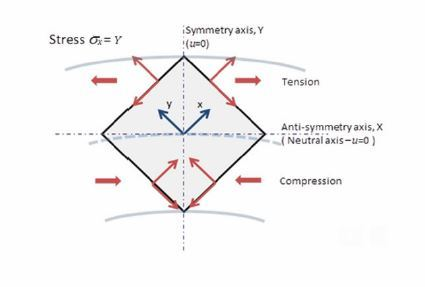### Submission by Husain J Al-Gahtani

From the given traction boundary conditions, one can obtain the following solution for the stresses: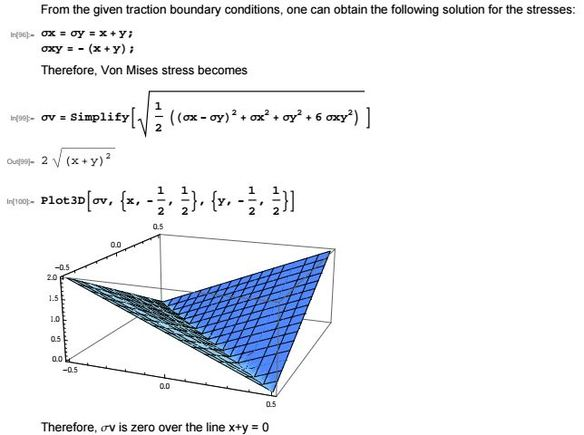In order to figure out how to restrain the plate such that rigid body motion is prevented ( a must for FEM solution), let us find strains then integrate them to get displacements (Es and Νs are E and Νs are for steel)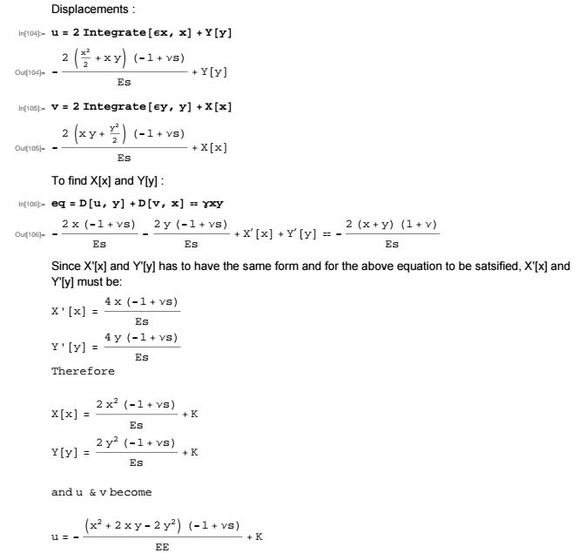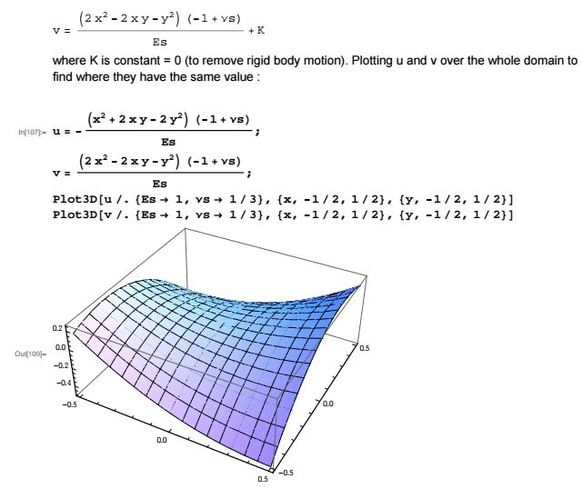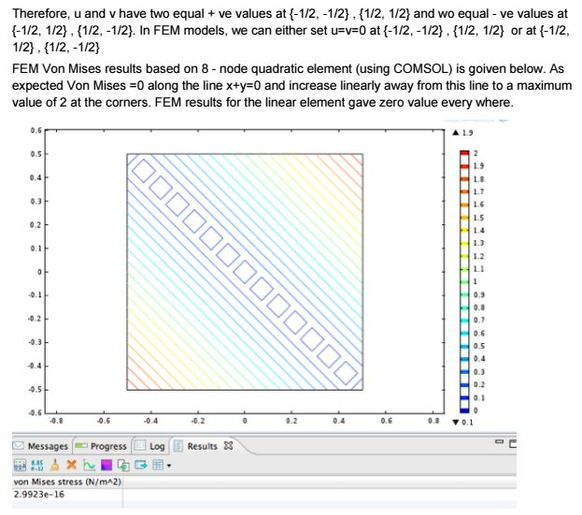Conclusion
One 8 - node quadratic element yields the exact solution. This is expected, since the exact solution for the displacements is quadratic and therefore one quadratic element is sufficient to interpolate the displacements exactly.

### Submission by Matt Watkins:

1. Von Mises Stress

Note: The problem statement asks for a “4 node element” and an “8 node element”. This is equivalent to asking for a solution with linear shape functions and a solution with quadratic shape functions. In StressCheck, the software used to solve this benchmark problem, the same element is used for both solutions and the user chooses the shape function order for the solution. For this problem, shape functions of order 1 (equivalent to the 4 node element) and of order 2 (equivalent to the 8 node element) were used for the solution.

1.1 Linear Solution

Von Mises stress for the linear solution (4 node element) is shown in Figure 1. The distribution of the von Mises stress over the element is, within numerical tolerance, zero. Therefore the von Mises stress is equal to zero throughout the entire element. The value reported at the center of the element is therefore zero.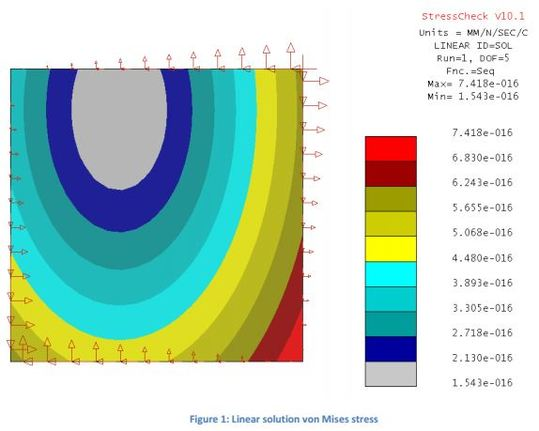Von Mises stress for the quadratic solution (8 node element) is shown in Figure 2. The distribution of the von Mises stress varies linearly across the element from 2 (lower left) to 0 (center) back to 2 (upper right). The value reported at the center of the element is again zero, even though the von Mises stress is not zero throughout the element.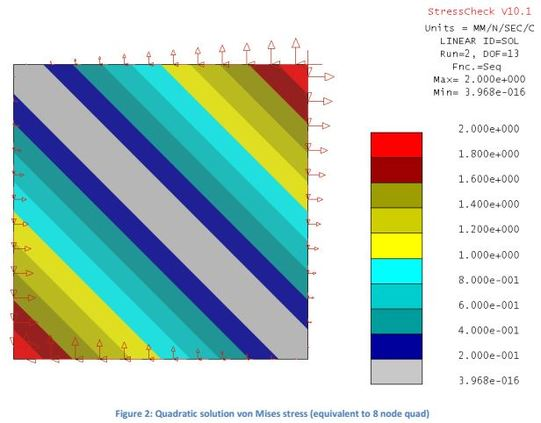Note on expected results from other software: The plot of von Mises stress in Figure 2 samples the finite element solution at 484 points (it is a grid of 22 by 22 points, which admittedly is overkill). This shows the exact von Mises stress throughout the element. Other FEA software products (ANSYS, Abaqus, Nastran, etc.) tend to interpolate nodal values of stress for contour plots instead of actually sampling the solution throughout the element. Therefore it is expected that most responders to this problem may report values around 1 Pa at the center of the element for the quadratic solution. A von Mises stress value of 1 Pa is incorrect, because nodal averaging at the center of the element does not produce the exact value of the von Mises stress at that point. Solving the problem with 4 or more elements will prove this point.

2. Which Value is Correct?
Both solutions report the value of the von Mises stress to be zero, which is correct at the center of the element but both solutions are misleading.

• The linear solution does not well-approximate the exact solution of the given problem. The solution is not converged. There is not sufficient resolution (number of degrees of freedom) to capture the solution. The reported stress value at the center of the element just happens to be correct, but reporting this value in an engineering analysis report would be insufficient because the exact von Mises stress varies throughout the element and the linear solution is not converged.

• The quadratic solution perfectly approximates the exact solution of the given problem. The solution is converged, meaning that there is sufficient resolution (number of degrees of freedom) to capture the solution. Convergence proof is given in section 2.1. The reported stress value at the center of the element is reported correctly as zero, although reporting this value in an engineering analysis report would be insufficient because the exact von Mises stress varies throughout the element.

2.1 Convergence Proof

As degrees of freedom are increased, the approximate solution produced by the finite element method approaches the exact solution of the posed problem. Four solutions are shown in Figure 3 with increasing resolution (increasing degrees of freedom, DOF). The linear and quadratic solutions required by the benchmark problem statement are shown in the top row. These solutions have 5 DOF and 13 DOF, respectively. As DOF are increased to 21 and then 31, shown in the bottom row, the solution does not change. Therefore, we have a good indication of convergence.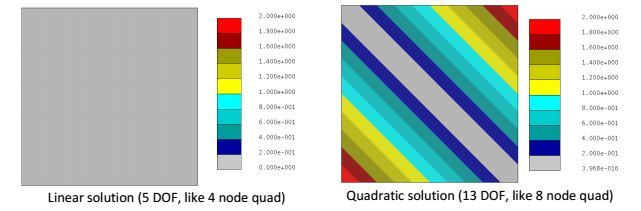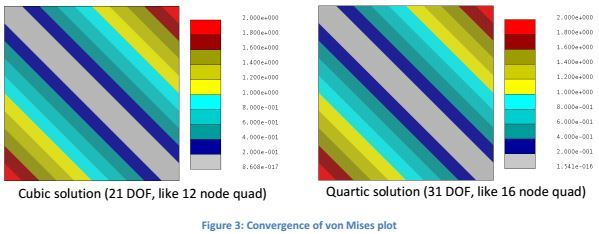A better metric for convergence for this problem would be the total potential energy value for each solution. The potential energy for the four solutions above is shown in Table 1. After the linear solution, the potential energy remains constant. Since the finite element method always minimizes the potential energy, a constant value of potential energy indicates that the computed solution is the exact solution, and therefore is correct (is converged).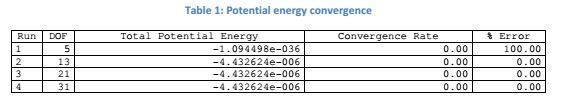3. Modeling Decisions

• Element: One quadrilateral element.
• Material: Plane stress (prescribed by problem statement) with arbitrary material properties chosen (does not impact stress solution).
• Loading: Tractions applied using spatial formulae on each element edge. The element with traction loading is shown in Figure 4 on the left. The spatial formulae are shown in the center. The loading for the selected element edge (red) is shown on the right.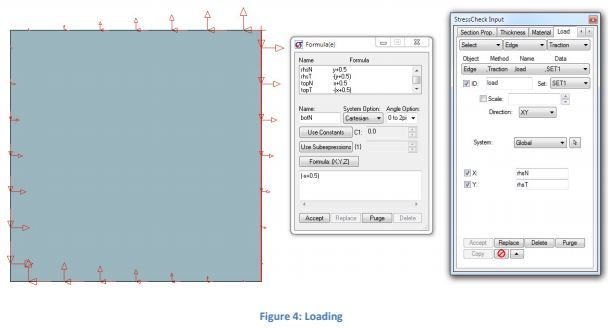• Constraints: The problem is in equilibrium, so only rigid body constraints are permissible whichmust not react any load. The choice of constrained nodes is arbitrary (does not impact stress solution). The constrained nodes chosen are shown in Figure 5.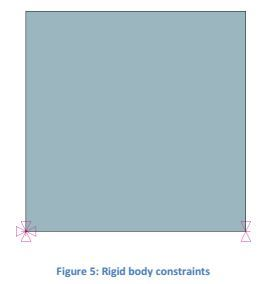4. FEA Software Details

• Software: StressCheck 10.1
• Total time to build problem and solve: 6 minutes

5 Terminology Note

The Benchmark Challenge problem terminology is not completely accurate. There is only one model (as described in the problem introduction and Figure 1 of the problem document) which is required to be solved using two particular discretizations: One element with shape functions of polynomial degree 1 and one element with shape functions of polynomial degree 2. The model (in this case based on the theory of elasticity with a particular shape and loading) and the solution of the model are defined independently of the discretization by which it is solved. The model is first solved with a poor approximation to its exact solution, and then the same model is solved with a higher resolution approximation which produces the exact solution. It makes for a good benchmark problem, though!

6 Supplement: H-Refinement

The exact solution of the posed problem was obtained with a single quad element and quadratic shape functions (p = 2). This section presents results for mesh refinement with linear shape functions (p =1, or more commonly: 4 node quads). The exact solution is obtained in the limit as the number of DOF goes to infinity, but convergence is very slow.
The following contour plots show the von Mises stress results by subdividing each element to increase DOF. Error is given for the maximum von Mises stress compared to the exact value which is 2.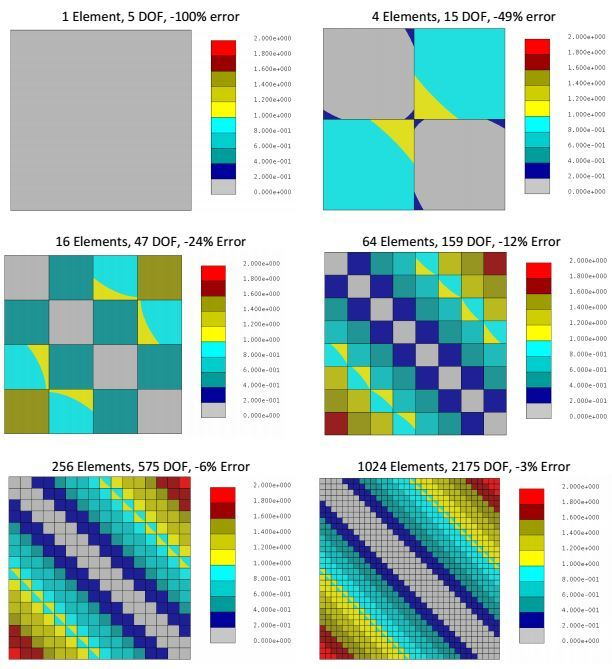The strain energy for each solution is shown in Table 2. Note strain energy depends on the material properties, so these should be taken as representative values.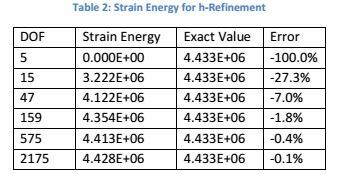Total time to build and solve 6 new solutions using mesh refinement: approximately 10-20 minutes with Stress Check.

To provide an honest non-software-dependent set of solutions, blending and averaging were turned off for the contour plots so that the data shown is exactly the data which is computed from the finite element solution on each element. Different FEA software may average computed data between elements differently, though in my experience this tends to make solution accuracy worse or harder to estimate.

Additionally, reduced integration was not used. Reduced integration attempts to cancel one error (error of approximation) with another error (error in integration). On some problems the errors may very well appear to cancel each other, but doing so precludes solution verification, and therefore should not be used in a benchmark problem setting.

6.1 Commentary

The exact solution of the posed problem was obtained with a single quad element with quadratic shape functions at 13 DOF. In contrast, a solution with linear shape functions has -3% error in maximum von Mises stress with 2175 DOF. This highlights the inefficiency of linear elements in general.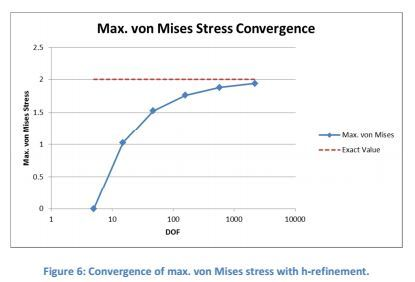Since the problem statement asked for the von Mises stress at the center of the element, which is at the minimum von Mises stress location, a convergence plot is also provided of the minimum stress.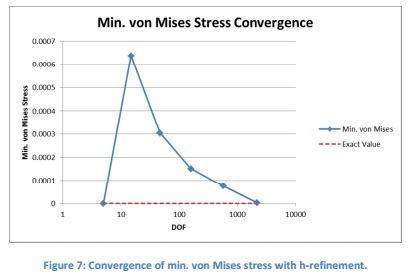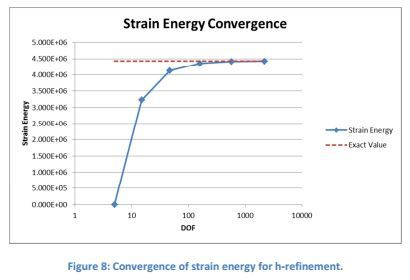Suggested questions to think about:

1. This is an extremely simple problem in the world of engineering. If it takes so many linear elements to well-approximate the exact solution, would linear elements be useful or efficient at capturing more complex solutions with practical consequence to engineering?

a. If time is not a factor, it doesn’t matter whether you use linear or quadratic or any other type of element. You can still well-approximate the exact solution – the much more important task is proving that your error of approximation is small.

b. Unfortunately, time is a factor. The ideal case is to quickly obtain a solution and quickly prove that the error of approximation (which is based on the mesh density, p-level, and mapping) is small enough.

2. How easy is it to obtain error estimates for solutions with FEA software?

a. With p-refinement in StressCheck the problem was built and solved with error estimates on all computed data in 6 minutes. Error estimates are provided automatically by the software.

b. With h-refinement the six computed solutions took 10-20 minutes (cumulative) to set up and solve, which is a factor of 2-3 on time. An external program (Excel) was used to calculate error estimates.

I hope you enjoyed these 3 thorough solutions offered as much as we did!
You can understand why we are now looking forward to receive the answers for the next installment.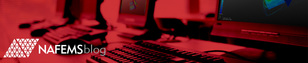# Request Further Information

It is often much simpler to discuss the benefits of NAFEMS membership and the options available with our dedicated development team.

If you would like one of our membership development managers to get in touch to discuss all aspects of NAFEMS, please fill out the form below, and we will talk to you soon.